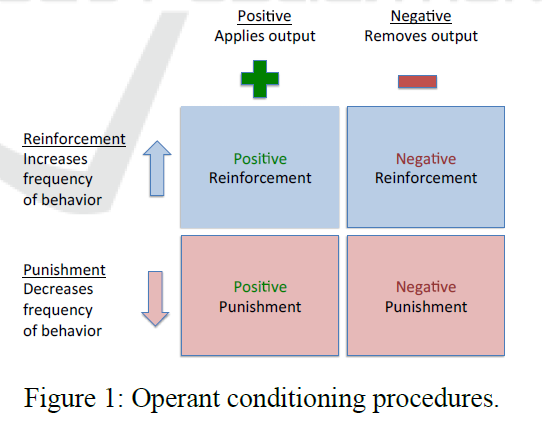# Quantum Probability in Operant Conditioning: Behavioral Uncertainty in Reinforcement Learning

Source: Deep Learning on Medium## Classical Probability V/S Quantum Probability

Next, the authors try to illustrate, how results differ in terms of operant conditioning vector space by applying quantum probability and classical probability techniques such as conjunction fallacy and the commutative property.

The magnitude of a projection depends on the angle between the corresponding subspaces. When the angle between subspaces is large a lot of probability amplitude is lost between successive projections.

Which results in || Pₐₚ|Ψ⟩||² < ||PₐₚPբᵣ|Ψ⟩||², that means, the direct projection to the Applies subspace (blue line) is less than the projection to the Applies subspace via the Frequency one (green line). which in classical probability is equivalent to Prob(Ap)< Prob(Ap & Fr), which is also impossible to achieve in classical probability. Because as the theory goes, The probability of two events occurring together is always less than or equal to the probability of either one occurring alone. Conversely, thinking that specific conditions are more probable than a single general one, is the well-known conjunction fallacy.

In Classical Probability, Prob(Fr & Ap) = Prob(Ap & Fr) but, in quantum probability theory, P(A)P(B) != P(B)P(A), therefore, the conjunction of incompatible questions fails commutativity. We see that Prob(Fr & Ap) = ||PₐₚPբᵣ|Ψ⟩||² is larger than Prob(Ap & Fr) = ||PբᵣPₐₚ|Ψ⟩||².

because in this case, we project from |Ψ⟩ to |Ap⟩ creating a large angle between them, and then from |Ap⟩ to |Fr⟩, we lose even more amplitude. In general, the smaller the angle between the subspaces for two incompatible questions the greater the relationship between the answers.

By projecting the state vector sequentially from one subspace to other there is a less loss of amplitude. This means, accepting one answer makes the other very likely or highly correlated as they say in classical probability.